# Standard #: MA.7.DP.1.1

This document was generated on CPALMS - www.cpalms.org

Determine an appropriate measure of center or measure of variation to summarize numerical data, represented numerically or graphically, taking into consideration the context and any outliers.

### Clarifications

Clarification 1: Instruction includes recognizing whether a measure of center or measure of variation is appropriate and can be justified based on the given context or the statistical purpose.

Clarification 2: Graphical representations are limited to histograms, line plots, box plots and stem-and-leaf plots.

Clarification 3: The measure of center is limited to mean and median. The measure of variation is limited to range and interquartile range.

### General Information

Subject Area: Mathematics (B.E.S.T.)
Strand: Data Analysis and Probability
Status: State Board Approved

### Terms from the K-12 Glossary

• Box Plot
• Data
• Histogram
• Interquartile Range (IQR)
• Line Plot
• Mean
• Measures of Center
• Measures of Variability
• Median
• Outlier
• Quartiles
• Range (of data set)
• Stem-and-Leaf Plot

### Vertical Alignment

Previous Benchmarks

Next Benchmarks

### Purpose and Instructional Strategies

In grade 6, students found and interpreted mean, median, mode and range, as well as determined and described how changes in data values impacted measures of center and variation. In grade 7, students determine an appropriate measure of center or measure of variation to summarize numerical data, taking into consideration the context and any outliers. Instruction builds on student knowledge from MA.6.DP.1.6. In grade 8, students will be introduced to numerical bivariate data and will depict it with line graphs and lines of fit. In high school, students will select an appropriate method to represent both univariate and bivariate numerical data, and interpret the different components in the display.
• The difference between range and interquartile range is just as important as, and very similar to, the difference between mean and median. In both cases, the difference has to do with whether or not one thinks outliers should be ignored (MTR.1.1, MTR.6.1).
• Outliers should be mostly ignored if a researcher is more interested in only the “typical” members of a population, as might be the case in politics or advertising. In these cases, the median is often the best choice as a measure of center, and the IQR is often the best choice as a measure of variation. These measures are little affected by outliers.
• Outliers should not be ignored if a researcher is concerned about the risks associated with “extreme” cases or concerned with the effects that outliers have on the average, as is the case in the insurance industry or in medical trials. In these cases, the mean is often a better choice than the median as a measure of center, and the range is better than the IQR as a measure of variation.
• All four of these measures are widely used in data analysis.
• Instruction focuses on identifying outliers qualitatively rather than quantitatively. To determine quantitatively if a data point is an outlier, a teacher may use the following definition. A data value is considered to be an outlier if it lies 1.5 times the IQR below
Q1, (Q1 − (1.5 · IQR)), or above Q3, (Q3 + (1.5 · IQR)).
• For example, the table below showcases a five-number summary of a data set.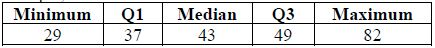Within the data set, the IQR is 12. To calculate if a value is an outlier, start with finding 1.5 · 12, which is 18. From there, a data value is considered an outlier if it is less than 37 − 18, or 19. It is also considered an outlier if it is greater than 49 + 18, or 67.
The maximum value in this data set is an outlier within the data set, though there could be additional values when you see the entire data set.
• Instruction includes cases where students are able to gather their own data for analysis (MTR.7.1).
• Instruction includes activities that require students to match graphs and explanations, or measures of center/variation and explanations prior to interpreting graphs based upon the appropriate measures of center or spread calculated (MTR.2.1, MTR.4.1).

### Common Misconceptions or Errors

• Some students may incorrectly calculate the measures of center and variation.
• Students may incorrectly believe all graphical displays are symmetrical. To address this misconception, students should use graphs of various shapes, including those with outliers, which will show this to be false. Start with small data sets related to familiar contexts to discuss how the data should be represented and to show how extreme values can alter the measures.
• Students may incorrectly identify data points as outliers.

### Strategies to Support Tiered Instruction

• Instruction includes opportunities for students to calculate the measures of center or the measures of variation for the initial and the changed data sets before comparing the impact of an outlier or an additional data point.
• Teacher provides examples of several visual displays or graphs to discuss the shapes of each one. Opportunities should be provided for students to see the various shapes with and without outliers so they can see that not all graphical displays are symmetrical.
• Teacher provides instruction on the definition of an outlier and interpretation on when to consider outliers (refer to the Instructional Strategies). Teacher provides examples of how outliers can be displayed within different types of data displays.
• Instruction includes co-creating a graphic organizer for measures of center and measures of variation and including the generalized impact of outliers on each.
• For example: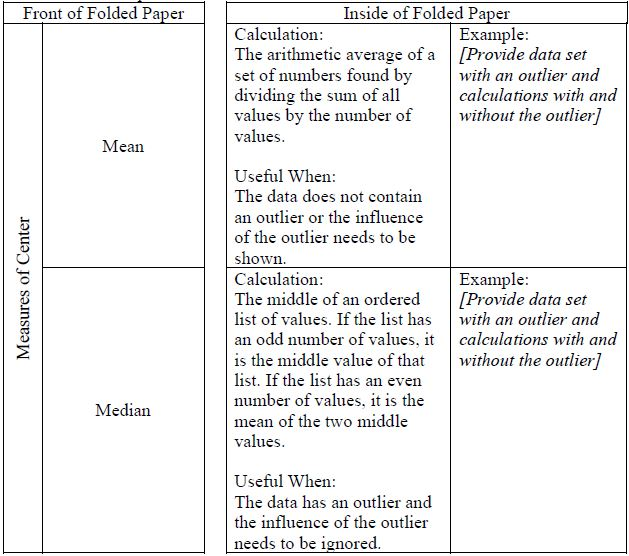Teacher Background Information
Unlike many elections for public office where a person is elected strictly based on the results of a popular vote (i.e., the candidate who earns the most votes in the election wins), in the United States, the election for President of the United States is determined by a process called the Electoral College. According to the National Archives, the process was established in the United States Constitution “as a compromise between election of the President by a vote in Congress and election of the President by a popular vote of qualified citizens.” (Archives -electoral college accessed July 1, 2021).
Each state receives an allocation of electoral votes in the process, and this allocation is determined by the number of members in the state’s delegation to the U.S. Congress. This number is the sum of the number of U.S. Senators that represent the state (always 2, per the Constitution) and the number of Representatives that represent the state in the U.S. House of Representatives (a number that is directly related to the state’s population of qualified citizens as determined by the US Census). Therefore the larger a state’s population of qualified citizens, the more electoral votes it has. Note: the District of Columbia (which is not a state) is granted 3 electoral votes in the process through the 23rd Amendment to the Constitution.

The following table shows the allocation of electoral votes for each state and the District of Columbia for the 2012, 2016 and 2020 presidential elections. (Archives -electoral college accessed July 1, 2021).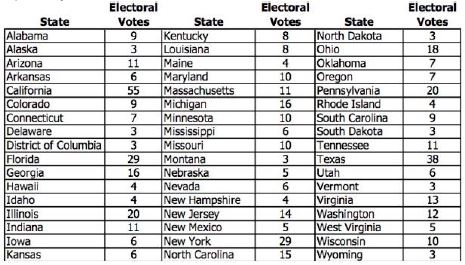• Part A. Which state has the most electoral votes? How many votes does it have?
• Part B. Based on the given information, which state has the second highest population of qualified citizens?
• Part C. Here is a line plot of the distribution.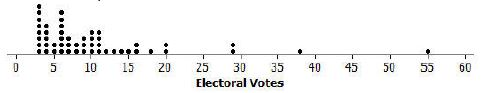• a. What is the shape of this distribution: symmetric or skewed?
• b. Imagine that someone you are speaking with is unfamiliar with these shape terms. Describe clearly and in the context of this data set what the shape description you have chosen means in terms of the distribution.
• Part D. Does the line plot lead you to think that any states are outliers in terms of their number of electoral votes? Explain your reasoning, and if you do believe that there are outlier values, identify the corresponding states.
• Part E. What measure of center (mean or median) would you recommend for describing this data set? Why did you choose this measure?
• Part F. Determine the value of the median for this data set (electoral votes).

### Instructional Items

Instructional Item 1
The household incomes of 15 families in a local neighborhood was recorded to the nearest \$1,000 in the table below.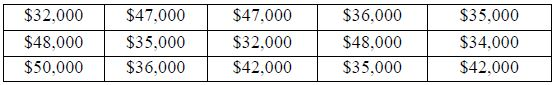Determine the most appropriate measure of center to describe this data set. What is the value of that measure?

Instructional Item 2
The household incomes of 15 families in a local neighborhood was recorded to the nearest \$1,000 in the table below.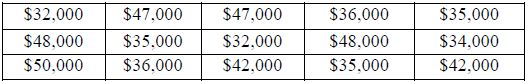At the end of the month, a new family is moving in whose household income is \$475,000. Determine the most appropriate measure of center to describe this data set. What is the value of that measure? Justify your choice.

*The strategies, tasks and items included in the B1G-M are examples and should not be considered comprehensive.

#### Related Courses

 Course Number1111 Course Title222 1205020: M/J Accelerated Mathematics Grade 6 (Specifically in versions: 2014 - 2015, 2015 - 2020, 2020 - 2022, 2022 and beyond (current)) 1205040: M/J Grade 7 Mathematics (Specifically in versions: 2014 - 2015, 2015 - 2022, 2022 and beyond (current)) 1204000: M/J Foundational Skills in Mathematics 6-8 (Specifically in versions: 2014 - 2015, 2015 - 2022, 2022 and beyond (current)) 7812020: Access M/J Grade 7 Mathematics (Specifically in versions: 2014 - 2015, 2015 - 2018, 2018 - 2019, 2019 - 2022, 2022 and beyond (current))

#### Related Access Points

 Access Point Number Access Point Title MA.7.DP.1.AP.1 Use context to determine the appropriate measure of center (mean or median) or range to summarize a numerical data set with 10 or fewer elements, represented numerically or graphically.

#### Formative Assessment

 Name Description Height Research Students are asked to describe a method for collecting data in order to estimate the average height of 12 year old boys in the U.S.